# Polarized algebraic variety

(diff) ← Older revision | Latest revision (diff) | Newer revision → (diff)

A pair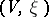, whereis a complex smooth variety (cf. Algebraic variety) over an algebraically closed field,is the class of some ample invertible sheaf (cf. Ample sheaf; Invertible sheaf) and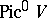is the connected component of the identity of the Abelian Picard scheme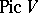. In the case whenis an Abelian variety, the concept of the degree of polarization of a polarized algebraic variety is also defined: It coincides with the degree of the isogeny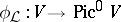determined by a sheaf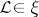, namely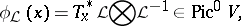where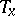is the morphism of translation by,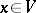. A polarization of degree one is called a principal polarization.

The concept of a polarized algebraic variety is closely connected with the concept of a polarized family of algebraic varieties. Letbe a family of varieties with base, that is,is a smooth projective morphism from the schemeto the Noetherian scheme, the fibres of which are algebraic varieties. The pair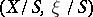, whereis the family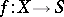with base, while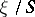is the class of the relatively-ample invertible sheafin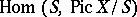modulo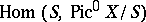, where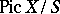is the relative Picard scheme, is called a polarized family.

The introduction of the concept of a polarized family and a polarized algebraic variety is required for the construction of moduli spaces of algebraic varieties (see Moduli theory). For example, there is no moduli space of all smooth algebraic curves of genus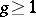, while for polarized curves there is such a space . One of the first questions connected with the concept of polarization of varieties is the question of simultaneous immersion in a projective space of polarized varieties with numerical invariants. Ifis contained as a fibre in a polarized familywith a connected baseand relatively-ample sheaf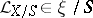, then does there exist a constantdepending only on the Hilbert polynomial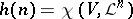such that for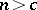the sheaves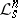with Hilbert polynomial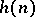and with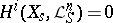for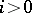, are very ample for all polarized algebraic varieties, where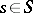? For smooth polarized algebraic varieties over an algebraically closed field of characteristicthe answer to this question is affirmative , while in the case of surfaces of principal type with the canonical polarization the constantis even independent of the Hilbert polynomial (see , ).

How to Cite This Entry:
Polarized algebraic variety. Encyclopedia of Mathematics. URL: http://encyclopediaofmath.org/index.php?title=Polarized_algebraic_variety&oldid=11345
This article was adapted from an original article by V.S. Kulikov (originator), which appeared in Encyclopedia of Mathematics - ISBN 1402006098. See original article